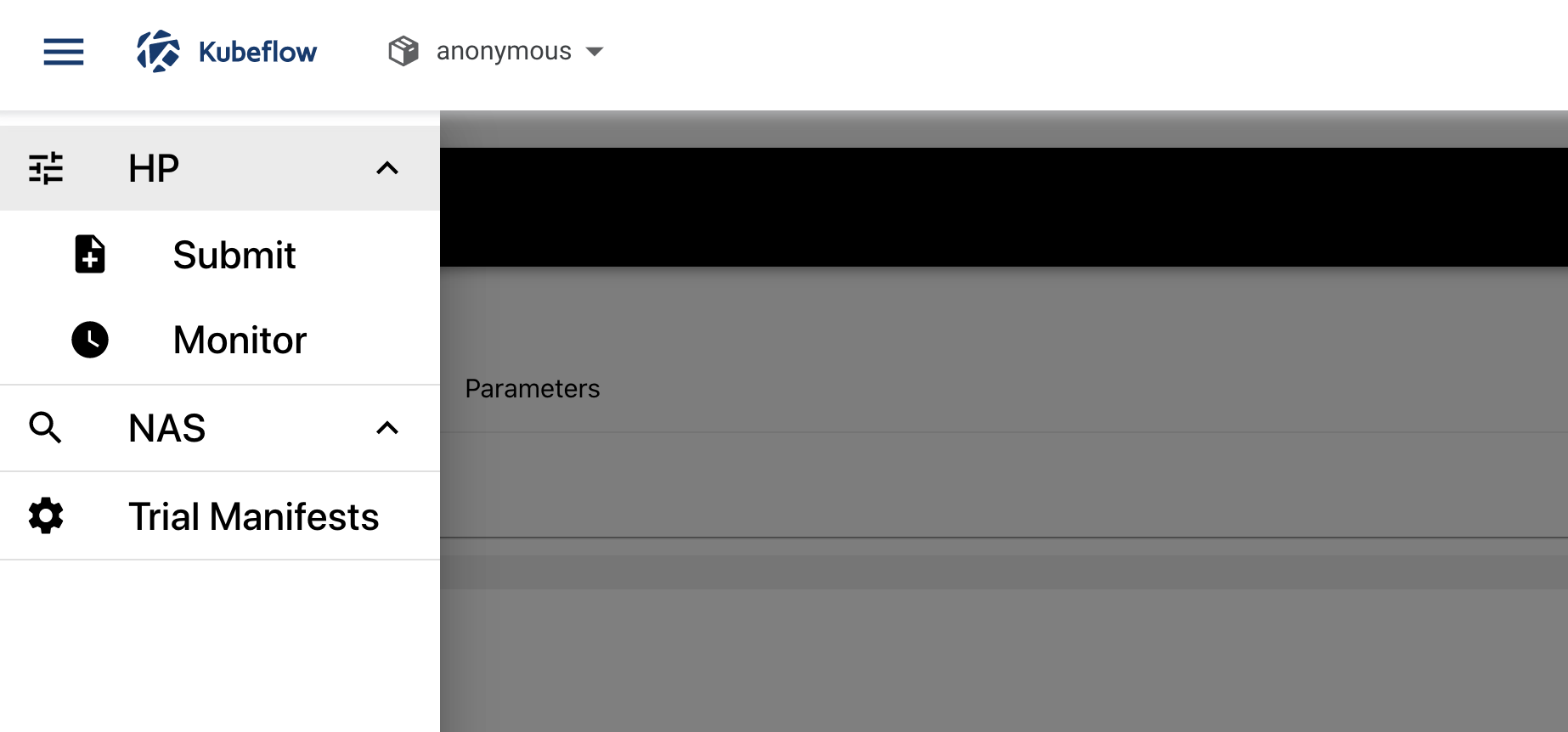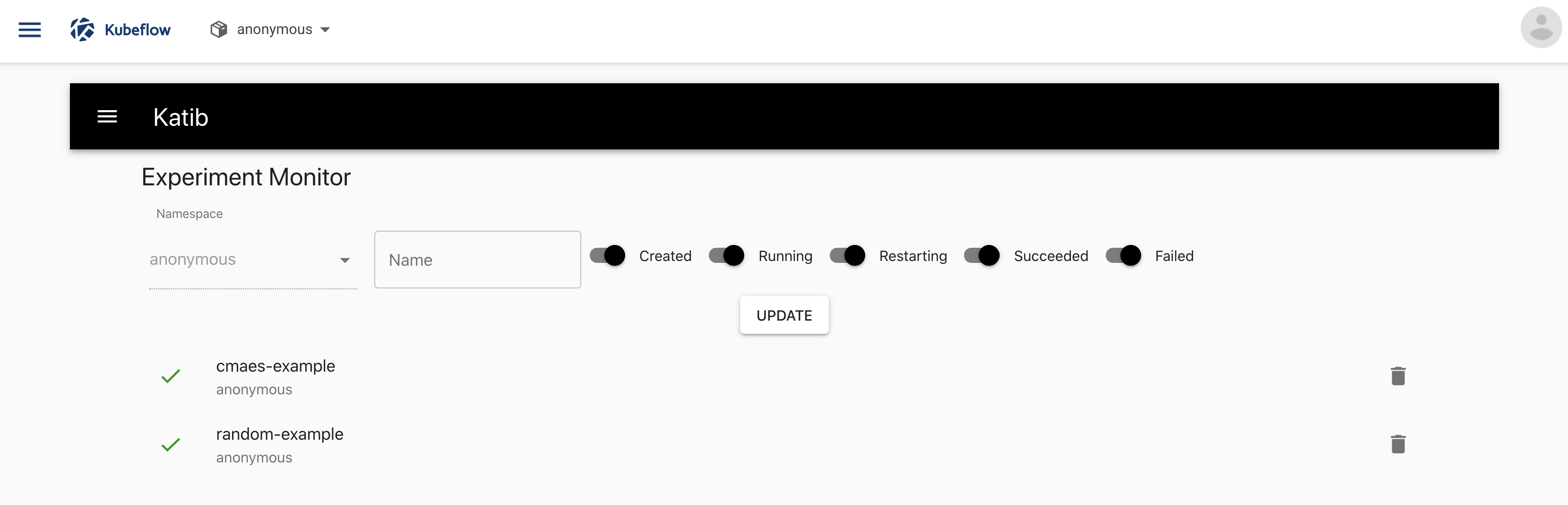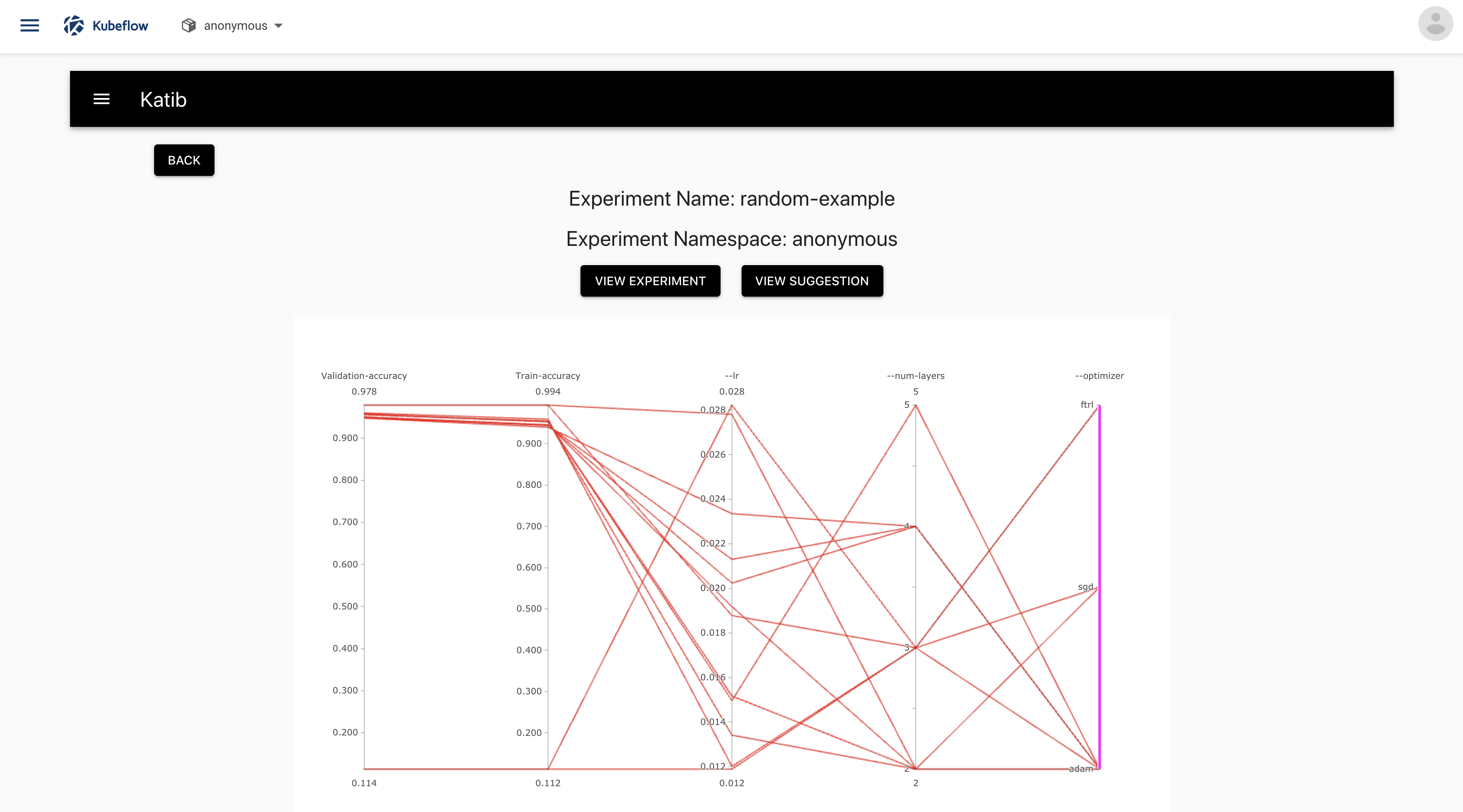# Running an experiment

How to configure and run a hyperparameter tuning or neural architecture search experiment in Katib

This page describes in detail how to configure and run a Katib experiment. The experiment can perform hyperparameter tuning or a neural architecture search (NAS) (alpha), depending on the configuration settings.

For an overview of the concepts involved, read the introduction to Katib.

## Packaging your training code in a container image

Katib and Kubeflow are Kubernetes-based systems. To use Katib, you must package your training code in a Docker container image and make the image available in a registry. See the Docker documentation and the Kubernetes documentation.

## Configuring the experiment

To create a hyperparameter tuning or NAS experiment in Katib, you define the experiment in a YAML configuration file. The YAML file defines the range of potential values (the search space) for the parameters that you want to optimize, the objective metric to use when determining optimal values, the search algorithm to use during optimization, and other configurations.

The list below describes the fields in the YAML file for an experiment. The Katib UI offers the corresponding fields. You can choose to configure and run the experiment from the UI or from the command line.

### Configuration spec

These are the fields in the experiment configuration spec:

• parameters: The range of the hyperparameters or other parameters that you want to tune for your ML model. The parameters define the search space, also known as the feasible set or the solution space. In this section of the spec, you define the name and the distribution (discrete or continuous) of every hyperparameter that you need to search. For example, you may provide a minimum and maximum value or a list of allowed values for each hyperparameter. Katib generates hyperparameter combinations in the range based on the hyperparameter tuning algorithm that you specify. See the `ParameterSpec` type.

• objective: The metric that you want to optimize. The objective metric is also called the target variable. A common metric is the model’s accuracy in the validation pass of the training job (validation-accuracy). You also specify whether you want Katib to maximize or minimize the metric.

Katib uses the `objectiveMetricName` and `additionalMetricNames` to monitor how the hyperparameters work with the model. Katib records the value of the best `objectiveMetricName` metric (maximized or minimized based on `type`) and the corresponding hyperparameter set in `Experiment.status`. If the `objectiveMetricName` metric for a set of hyperparameters reaches the `goal`, Katib stops trying more hyperparameter combinations.

You can run experiment without specifying the `goal`. In that case, Katib runs experiment until corresponding succeeded trials reaches `maxTrialCount`. `maxTrialCount` parameter is described bellow.

See the `ObjectiveSpec` type.

• algorithm: The search algorithm that you want Katib to use to find the best hyperparameters or neural architecture configuration. Examples include random search, grid search, Bayesian optimization, and more. See the search algorithm details below.

• trialTemplate: The template that defines the trial. You must package your ML training code into a Docker image, as described above. You must configure the model’s hyperparameters either as command-line arguments or as environment variables, so that Katib can automatically set the values in each trial.

You can use one of the following job types to train your model:

See the `TrialTemplate` type. The template uses the Go template format.

You can define the job in raw string format or you can use a ConfigMap. Here is an example how to create ConfigMap with trial templates.

• parallelTrialCount: The maximum number of hyperparameter sets that Katib should train in parallel.

• maxTrialCount: The maximum number of trials to run. This is equivalent to the number of hyperparameter sets that Katib should generate to test the model.

• maxFailedTrialCount: The maximum number of failed trials before Katib should stop the experiment. This is equivalent to the number of failed hyperparameter sets that Katib should test. If the number of failed trials exceeds `maxFailedTrialCount`, Katib stops the experiment with a status of `Failed`.

• metricsCollectorSpec: A specification of how to collect the metrics from each trial, such as the accuracy and loss metrics. See the details of the metrics collector below.

• nasConfig: The configuration for a neural architecture search (NAS). Note: NAS is currently in alpha with limited support. You can specify the configurations of the neural network design that you want to optimize, including the number of layers in the network, the types of operations, and more. See the `NasConfig` type.

• graphConfig: The graph config that defines structure for a directed acyclic graph of the neural network. You can specify number of layers, `input_sizes` for input layer and `output_sizes` for output layer. See the `GraphConfig` type.

• operations: The range of operations that you want to tune for your ML model. For each neural network layer NAS algorithm selects one of the operation to build neural network. Each operation has sets of parameters which described above. See the `Operation` type.

You can find all NAS examples here.

• resumePolicy: Experiment resume policy. If experiment was succeeded because `maxTrialCount` was reached, you can resume it by increasing `maxTrialCount`. Specify `resumePolicy: LongRunning`, if you want to use this feature. If you don’t need to resume experiment, specify `resumePolicy: Never`. In that case, suggestion resources will be deleted and experiment can’t be resumed. By default all experiments have `resumePolicy: LongRunning` parameter. See the `ResumePolicy` type.

Background information about Katib’s `Experiment` type: In Kubernetes terminology, Katib’s `Experiment` type is a custom resource (CR). The YAML file that you create for your experiment is the CR specification.

### Search algorithms in detail

Katib currently supports several search algorithms. See the `AlgorithmSpec` type.

More algorithms are under development. You can add an algorithm to Katib yourself. See the guide to adding a new algorithm and the developer guide.

The algorithm name in Katib is `grid`.

Grid sampling is useful when all variables are discrete (as opposed to continuous) and the number of possibilities is low. A grid search performs an exhaustive combinatorial search over all possibilities, making the search process extremely long even for medium sized problems.

Katib uses the Chocolate optimization framework for its grid search.

The algorithm name in Katib is `random`.

Random sampling is an alternative to grid search, useful when the number of discrete variables to optimize is large and the time required for each evaluation is long. When all parameters are discrete, random search performs sampling without replacement. Random search is therefore the best algorithm to use when combinatorial exploration is not possible. If the number of continuous variables is high, you should use quasi random sampling instead.

Katib uses the Hyperopt, Goptuna or Chocolate optimization framework for its random search.

Katib supports the following algorithm settings:

Setting name Description Example
random_state [int]: Set `random_state` to something other than None for reproducible results. 10

#### Bayesian optimization

The algorithm name in Katib is `bayesianoptimization`.

The Bayesian optimization method uses gaussian process regression to model the search space. This technique calculates an estimate of the loss function and the uncertainty of that estimate at every point in the search space. The method is suitable when the number of dimensions in the search space is low. Since the method models both the expected loss and the uncertainty, the search algorithm converges in a few steps, making it a good choice when the time to complete the evaluation of a parameter configuration is long.

Katib uses the Scikit-Optimize or Chocolate optimization framework for its Bayesian search. Scikit-Optimize is also known as `skopt`.

Katib supports the following algorithm settings:

Setting Name Description Example
base_estimator [“GP”, “RF”, “ET”, “GBRT” or sklearn regressor, default=“GP”]: Should inherit from `sklearn.base.RegressorMixin`. The `predict` method should have an optional `return_std` argument, which returns `std(Y | x)` along with `E[Y | x]`. If `base_estimator` is one of [“GP”, “RF”, “ET”, “GBRT”], the system uses a default surrogate model of the corresponding type. See more information in the skopt documentation. GP
n_initial_points [int, default=10]: Number of evaluations of `func` with initialization points before approximating it with `base_estimator`. Points provided as `x0` count as initialization points. If `len(x0) < n_initial_points`, the system samples additional points at random. See more information in the skopt documentation. 10
acq_func [string, default=`"gp_hedge"`]: The function to minimize over the posterior distribution. See more information in the skopt documentation. gp_hedge
acq_optimizer [string, “sampling” or “lbfgs”, default=“auto”]: The method to minimize the acquisition function. The system updates the fit model with the optimal value obtained by optimizing `acq_func` with `acq_optimizer`. See more information in the skopt documentation. auto
random_state [int]: Set `random_state` to something other than None for reproducible results. 10

#### Hyperband

The algorithm name in Katib is `hyperband`.

Katib supports the Hyperband optimization framework. Instead of using Bayesian optimization to select configurations, Hyperband focuses on early stopping as a strategy for optimizing resource allocation and thus for maximizing the number of configurations that it can evaluate. Hyperband also focuses on the speed of the search.

#### Tree of Parzen Estimators (TPE)

The algorithm name in Katib is `tpe`.

Katib uses the Hyperopt or Goptuna optimization framework for its TPE search.

This method provides a forward and reverse gradient-based search.

#### Covariance Matrix Adaptation Evolution Strategy (CMA-ES)

The algorithm name in Katib is `cmaes`.

Katib uses the Goptuna optimization framework for its CMA-ES search.

The Covariance Matrix Adaptation Evolution Strategy is a stochastic derivative-free numerical optimization algorithm for optimization problems in continuous search spaces.

Katib supports the following algorithm settings:

Setting name Description Example
random_state [int]: Set `random_state` to something other than None for reproducible results. 10
sigma [float]: Initial standard deviation of CMA-ES. 0.001

#### Neural Architecture Search based on ENAS

The algorithm name in Katib is `enas`.

This NAS algorithm ENAS-based. Currently, it doesn’t support parameter sharing.

Katib supports the following algorithm settings:

Setting Name Type Default value Description
controller_hidden_size int 64 RL controller lstm hidden size. Value must be >= 1.
controller_temperature float 5.0 RL controller temperature for the sampling logits. Value must be > 0. Set value to "None" to disable it in controller.
controller_tanh_const float 2.25 RL controller tanh constant to prevent premature convergence. Value must be > 0. Set value to "None" to disable it in controller.
controller_entropy_weight float 1e-5 RL controller weight for entropy applying to reward. Value must be > 0. Set value to "None" to disable it in controller.
controller_baseline_decay float 0.999 RL controller baseline factor. Value must be > 0 and <= 1.
controller_learning_rate float 5e-5 RL controller learning rate for Adam optimizer. Value must be > 0 and <= 1.
controller_skip_target float 0.4 RL controller probability, which represents the prior belief of a skip connection being formed. Value must be > 0 and <= 1.
controller_skip_weight float 0.8 RL controller weight of skip penalty loss. Value must be > 0. Set value to "None" to disable it in controller.
controller_train_steps int 50 Number of RL controller training steps after each candidate run. Value must be >= 1.
controller_log_every_steps int 10 Number of RL controller training steps before logging it. Value must be >= 1.

• Information in the Katib repository on Efficient Neural Architecture Search.
• As a ENAS example, see the YAML file for the enas-example-gpu. The example aims to show all the possible operations. Due to the large search space, the example is not likely to generate a good result.

#### Differentiable Architecture Search (DARTS)

The algorithm name in Katib is `darts`.

Currently, you can’t view results of this algorithm in the Katib UI and you can run experiment only on single GPU.

Katib supports the following algorithm settings:

Setting Name Type Default value Description
num_epochs int 50 Number of epochs to train model
w_lr float 0.025 Initial learning rate for training model weights. This learning rate annealed down to `w_lr_min` following a cosine schedule without restart.
w_lr_min float 0.001 Minimum learning rate for training model weights.
w_momentum float 0.9 Momentum for training training model weights.
w_weight_decay float 3e-4 Training model weight decay.
w_grad_clip float 5.0 Max norm value for clipping gradient norm of training model weights.
alpha_lr float 3e-4 Initial learning rate for alphas weights.
alpha_weight_decay float 1e-3 Alphas weight decay.
batch_size int 128 Batch size for dataset.
init_channels int 16 Initial number of channels.
print_step int 50 Number of training or validation steps before logging it.
num_nodes int 4 Number of DARTS nodes.
stem_multiplier int 3 Multiplier for initial channels. It is used in first stem cell.

### Metrics collector

In the `metricsCollectorSpec` section of the YAML configuration file, you can define how Katib should collect the metrics from each trial, such as the accuracy and loss metrics. See the `MetricsCollectorSpec` type

Your training code can record the metrics into `stdout` or into arbitrary output files. Katib collects the metrics using a sidecar container. A sidecar is a utility container that supports the main container in the Kubernetes Pod.

To define the metrics collector for your experiment:

1. Specify the collector type in the `collector` field. Katib’s metrics collector supports the following collector types:

• `StdOut`: Katib collects the metrics from the operating system’s default output location (standard output).
• `File`: Katib collects the metrics from an arbitrary file, which you specify in the `source` field.
• `TensorFlowEvent`: Katib collects the metrics from a directory path containing a tf.Event. You should specify the path in the `source` field.
• `Custom`: Specify this value if you need to use custom way to collect metrics. You must define your custom metrics collector container in the `collector.customCollector` field.
• `None`: Specify this value if you don’t need to use Katib’s metrics collector. For example, your training code may handle the persistent storage of its own metrics.
2. Specify the metrics output location in the `source` field. See const for default values.

3. Write code in your training container to print metrics in the format specified in the `metricsCollectorSpec.source.filter.metricsFormat` field. The default format is `([\w|-]+)\s*=\s*((-?\d+)(\.\d+)?)`. Each element is a regular expression with two subexpressions. The first matched expression is taken as the metric name. The second matched expression is taken as the metric value.

For example, using the default metrics format, if the name of your objective metric is `loss` and the metrics are `recall` and `precision`, your training code should print the following output:

``````epoch 1:
loss=0.3
recall=0.5
precision=0.4

epoch 2:
loss=0.2
recall=0.55
precision=0.5
``````

## Running the experiment

You can run a Katib experiment from the command line or from the Katib UI.

### Running the experiment from the command line

You can use kubectl to launch an experiment from the command line:

``````kubectl apply -f <your-path/your-experiment-config.yaml>
``````

For example, run the following command to launch an experiment using the random algorithm example:

``````kubectl apply -f https://raw.githubusercontent.com/kubeflow/katib/master/examples/v1alpha3/random-example.yaml
``````

Check the experiment status:

``````kubectl -n kubeflow describe experiment <your-experiment-name>
``````

For example, to check the status of the random algorithm example:

``````kubectl -n kubeflow describe experiment random-example
``````

### Running the experiment from the Katib UI

Instead of using the command line, you can submit an experiment from the Katib UI. The following steps assume you want to run a hyperparameter tuning experiment. If you want to run a neural architecture search, access the NAS section of the UI (instead of the HP section) and then follow a similar sequence of steps.

To run a hyperparameter tuning experiment from the Katib UI:

1. Follow the getting-started guide to access the Katib UI.

3. Open the Katib menu panel on the left, then open the HP section and click Submit:4. You should see tabs offering you the following options:

• YAML file: Choose this option to supply an entire YAML file containing the configuration for the experiment.• Parameters: Choose this option to enter the configuration values into a form.View the results of the experiment in the Katib UI:

1. Open the Katib menu panel on the left, then open the HP section and click Monitor:2. You should see the list of experiments:3. Click the name of your experiment. For example, click random-example.

4. You should see a graph showing the level of validation and train accuracy for various combinations of the hyperparameter values. For example, the graph below shows learning rate, number of layers, and optimizer:5. Below the graph is a list of trials that ran within the experiment. Click a trial name to see the trial data.

## Next steps

• See how to run the random algorithm and other Katib examples in the getting-started guide.

• For an overview of the concepts involved in hyperparameter tuning and neural architecture search, read the introduction to Katib.

• For a detailed instruction of the Katib Configuration file, read the Katib config page.

• See how you can change installation of Katib component in the environment variables guide.